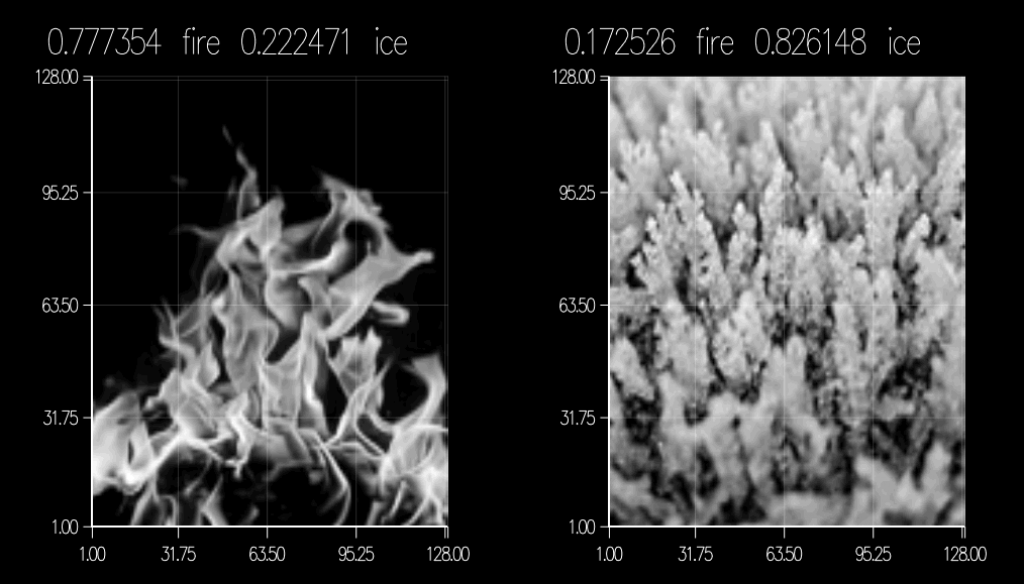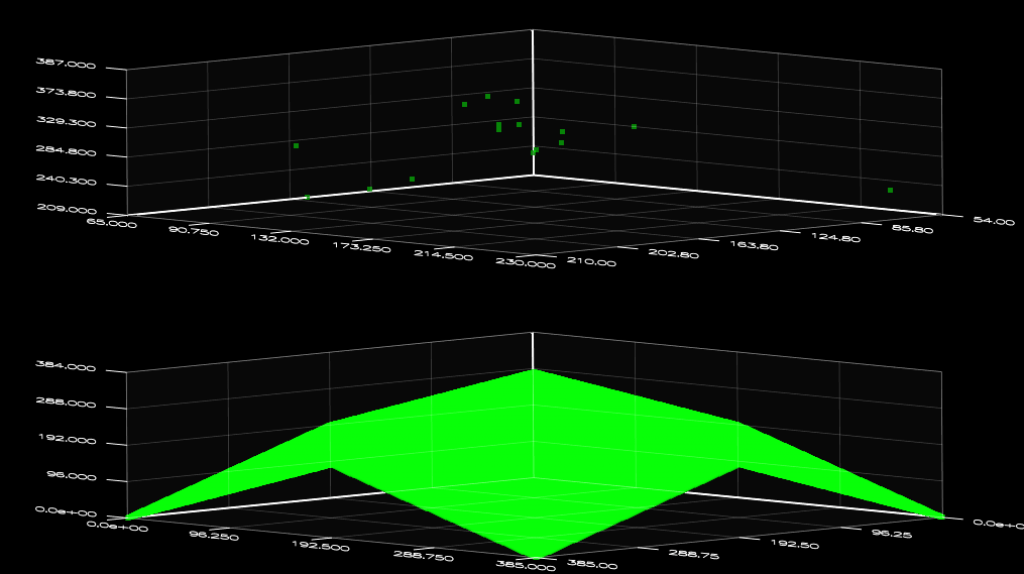# ArrayFire Examples (Part 5 of 8) – Machine Learning

This is the fifth in a series of posts looking at our current ArrayFire examples. The code can be compiled and run from `arrayfire/examples/` when you download and install the ArrayFire library. Today we will discuss the examples found in the `machine_learning/` directory.

In these examples, my machine has the following configuration:

```ArrayFire v1.9 (build XXXXXXX) by AccelerEyes (64-bit Mac OSX)
CUDA toolkit 5.0, driver 304.54
GPU0 GeForce GT 560M, 1024 MB, Compute 3.0 (single,double)
Display Device: GPU0 GeForce GT 650M
Memory Usage: 245 MB free (1024 MB total)...```

1. K-Means Clustering – kmeans.cppFigure 1

This is an example of K-Means Clustering Algorithm. K-Means Clustering Algorithm is a data mining technique that partitions the given data into groups by their similarities. All you need to know from this example is that this algorithm will repeatedly run through many loops to stabilize the clusters. Again, ArrayFire has this beautiful tool called GFOR loop that handles any matrix array arithmetic and transformation in parallel. This increases the speed of both the cluster stabilization and k-means computation in this example.

``` 35        gfor(array i, num_uniq) {    // current classification
36             array diffs = abs(hist_idx(i) - means_vec);
37             array val, idx;
38             min(val, idx, diffs);
39             hist_counts(hist_idx(i)) = idx;
40         }
..
57     gfor(array i, k) { // pixel differences from computed means
58         vol(span, span, i) = abs(data - means_vec(i));
59     }```

Just to verify the speed improvement in computation with ArrayFire again, I made a simple change in the code to find the elapsed times in FOR loop (left below) and GFOR loop (right below) as following.

``` FOR Loop                                               GFOR Loop
36  clock_t begin = clock();                           47  clock_t begin = clock();
37  for(int i=0; i<num_uniq; i++){                     48  gfor(array i, num_uniq) {    // current classification
38    array diffs = abs(hist_idx(i) - means_vec);      49    array diffs = abs(hist_idx(i) - means_vec);
39    array val, idx;                                  50    array val, idx;
40    min(val, idx, diffs);                            51    min(val, idx, diffs);
41    hist_counts(hist_idx(i)) = idx;                  52    hist_counts(hist_idx(i)) = idx;
42  }                                                  53  }
43                                                     54
44  clock_t end = clock();                             55  clock_t end = clock();
45  double t =  double(end - begin) / CLOCKS_PER_SEC;  56  double t =  double(end - begin) / CLOCKS_PER_SEC;
46  printf("Elapsed Time: %f secn", t);               57  printf("Elapsed Time: %f secn", t);```

In result, each GFOR loop was approximately 200x faster than each FOR loop as shown below. Since there were a total of twelve loops in this example, ArrayFire has performed almost 2000x faster in time. If there were more loops performed, you can imagine how much time we would save with ArrayFire.

```FOR Loop                                    GFOR Loop
Elapsed Time: 0.016808 sec                  Elapsed Time: 0.002231 sec
Elapsed Time: 0.014868 sec                  Elapsed Time: 0.000676 sec
Elapsed Time: 0.053122 sec                  Elapsed Time: 0.000088 sec
Elapsed Time: 0.048089 sec                  Elapsed Time: 0.000074 sec
Elapsed Time: 0.048284 sec                  Elapsed Time: 0.000074 sec
Elapsed Time: 0.033541 sec                  Elapsed Time: 0.000075 sec
Elapsed Time: 0.013796 sec                  Elapsed Time: 0.000068 sec
Elapsed Time: 0.029183 sec                  Elapsed Time: 0.000068 sec
Elapsed Time: 0.011643 sec                  Elapsed Time: 0.000068 sec
Elapsed Time: 0.011273 sec                  Elapsed Time: 0.000067 sec
Elapsed Time: 0.026709 sec                  Elapsed Time: 0.000068 sec
Elapsed Time: 0.010750 sec                  Elapsed Time: 0.000068 sec```

The number of loops in this example was actually arbitrary because stabling the cluster could take longer than expected depending on the situation; thus it was a great example to evaluate the performance of ArrayFire. In the result of my experiment, ArrayFire was again proven to provide big performance benefits within loops.

2. Neural Network – neuralnetwork.cppFigure 2

This is an example of Neural Network algorithm. Neural Network algorithm (also known as Artificial Neural Network) is another data mining technique that is inspired by biological neural network system. I won’t talk about the details of this algorithm, but let’s first take a look at the three following images.These three layers are used as an input for our algorithm. The layers first get joined in a single matrix array by JOIN function after flattened into a column vector by FLAT function. The algorithm contains a “train” procedure with a given number of iteration time (200 iterations in this example). During this iteration process, the algorithm determines errors for each layer and corrects them to train (or learn) the most optimized weight for each pixel using gradient. As shown Figure 2, the example loads another image to compare with the optimized pixel values after the train procedure has completed. It finally prints out the result of numbers that indicates the difference between the image (in Figure 2) and trained weights.

``` 80     array samples = join(0,
84     samples = join(0,
85                    samples,
89```

Normally, this algorithm has to be done with the massive number of iteration for each pixel in all three layers. ArrayFire is, again, convenient in this algorithm; instead of having three layers independently, ArrayFire uses JOIN function to combine all the layers into one single matrix array. This way, ArrayFire reduces all the matrix iterations and maximizes the efficiency of parallel matrix arithmetics (such as MATMUL, POW, and TILE). Basically, this example ended up not requiring any matrix iteration by using ArrayFire (but still requires only the iterations for the number of train, which must be done without any trick).

3. Principal Component Analysis – pca.cppFigure 3

This is an example of Principal Component Analysis. Principal Component Analysis is yet another data mining technique for finding unique patterns in high-dimensional data space. This technique is often found in applications for face recognition and image compression. The basic idea is to reduce the high-dimensional data space to a lower dimension in order to have a simpler representation of data set; so, it is easier to handle the data set as well as to plot them in a human readable dimensional space (three-dimension in our example).

``` 20     // data normalization
21     array means = mean(data);
22     array stdvs = stdev(data);
23     array sdata = (data - tile(means, n, 1)) / tile(stdvs, n, 1);
24
25     // decomposition
26     array V, D, E;
27     eigen(V, D, cov(sdata));
28     E = diag(D);
29     array val, idx;
30     sort(val, idx, -1*E);
31     array comps = matmul(sdata, V); // principal components
..
68         fig("sub",2,1,1); fig("title","Input");
69         plot3(data(span,1), data(span,2), data(span,3));
70
71         fig("sub",2,1,2); fig("title","14D->3D PCA");
72         plot3(proj(span,1), proj(span,2), proj(span,3));
73
74         fig("draw");
75     }```

For this purpose, this algorithm consists of various matrix transformations such as Covariance (COV), Standard Deviation (STDEV), Eigenvalues (EIGEN), Means (MEAN), Multiplication (MATMUL), and Sort (SORT). The ArrayFire library already has these functions available for user’s usage. ArrayFire certainly accelerates the speed of these matrix transformations as we have shown in previous examples. Finally, this example uses PLOT3 function to plot the final data set in three-dimensional space for visualization as shown in Figure 3.

4. Genetic Algorithm – geneticalgorithm.cppFigure 4

This is an example of Genetic Algorithm. Genetic Algorithm is the last data mining technique in this post. This algorithm is inspired by the concept of neurological development as in learning through repetition over time. Therefore, a good model to describe this algorithm is the brain, which habitually tries and learns to achieve the optimal answer. The algorithm is a powerful tool for many applications, but the steps are simple enough.

``` 33 array selectFittest() {
34     //pick top 50% fittest
35     array sorted = sort(samplez);
36     array indices = where(samplez >= sorted.row(nsamples / 2));
37
38     if (indices.elements() > nsamples / 2) {
39         indices = indices.rows(indices.elements() - nsamples / 2, indices.elements() - 1);
40     }
41
42     return indices;
43 }```

First, the algorithm selects only the top 50% fittest data elements (potential candidates close to the answer) from the initial data set. This may require to create a new data structure with unknown size and to check each element under the given condition in order to collect only the wanted data. Normally, the performance can be really slow, because it has to resize the data structure and check with the condition. To accomplish this procedure more efficient and faster, ArrayFire has WHERE function that collects the elements that satisfy the specific requirements, of course, in a parallel manner, and forms a new matrix array with the collected elements.

``` 52     //Divide selection in two
53     array parentsx1 = parentsx.rows(0, parentsx.elements() / 2 - 1);
54     array parentsx2 = parentsx.rows(parentsx.elements() / 2, parentsx.elements() - 1);
55     array parentsy1 = parentsy.rows(0, parentsy.elements() / 2 - 1);
56     array parentsy2 = parentsy.rows(parentsy.elements() / 2, parentsy.elements() - 1);
..
63     //Create children as the cross between two parents
66

After the new data set has been created with the fittest elements, secondly, the algorithm divides the elements in two parent groups and uses the crossover points to create children (You don’t need to know how to calculate the crossover points in this example; all you need to know is that the algorithm uses the crossover points to generate children for the next generation). For this procedure, the algorithm uses many element-wise arithmetic operations. Without ArrayFire library, this must take a long annoying waiting time to finish these same computations repeatedly. But, since the same equations will be applied on every element, we can simply use Element-wise arithmetics, already built in ArrayFire library, to apply the maths on all the elements in a parallel manner.

``` 70     //Join two new sets
71     samplex = join(0, children_x1, children_x2);
72     sampley = join(0, children_y1, children_y2);
..
83     samplex = join(0, samplex, mutantx);
84     sampley = join(0, sampley, mutanty);```

Thirdly, the algorithm performs JOIN to combine all the children in one single matrix array indicating itself as the current generation. In the meantime, it generates mutant elements by purposely masking random bits; mutants are formed to possibly arrive closer to the answer by random chance (Again, you don’t need to know much detail about the Mutant either if you are only here for learning ArrayFire). Lastly, it updates current data set with the new generation data set and plot into three-dimensional space for display. With our ArrayFire library, the algorithm is now capable of executing these heavy procedures very quickly in real time.

Machine Learning is really a technique that requires lots of digging, collecting, organizing, and optimizing the massive data sets. If you ever felt it slow, download the ArrayFire library and accelerate your performance today!

Posts in this series: